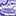# New to Qlik Sense

If you’re new to Qlik Sense, start with this Discussion Board and get up-to-speed quickly.

Announcements
cancel
Showing results for
Did you mean:Contributor II

## Return first month of the Fiscal Year regardless of month chosen

Hello everyone,

Quick q, I am trying to create a code so that regardless of the month you select within your fiscal year, it will always return the first month of that fiscal year (April). So if I selected October 2018, it will return the data for April 2018. Does anyone know how to write the code for this?

Currently my master calendar looks like this:

MasterCalendar:

TempDate as Date,

Year(TempDate) as Year,

Month(TempDate) as Month,

If(Month(TempDate)=\$(vFM),1,0) as AprilFlag,

YeartoDate(TempDate)*-1 as CurYTDFlag,

YeartoDate(TempDate,-1)*-1 as LastYTDFlag,

Year(TempDate) + If(Month(TempDate)>=\$(vFM),1,0) as [Fiscal Year],

Date(monthstart(TempDate), 'YYYYMM') as YearMonth,

Year(TempDate) + If(Month(TempDate)=\$(vFM),1,0) &

(Month(TempDate)= 'Apr') as YearAprilFlag,

Year(TempDate) + If(Month(TempDate)>=\$(vFM),1,0) &

if(Month(TempDate)= 'Apr',01,

if(Month(TempDate)= 'May',02,

if(Month(TempDate)= 'Jun',03,

if(Month(TempDate)= 'Jul',04,

if(Month(TempDate)= 'Aug',05,

if(Month(TempDate)= 'Sep',06,

if(Month(TempDate)= 'Oct',07,

if(Month(TempDate)= 'Nov',08,

if(Month(TempDate)= 'Dec',09,

if(Month(TempDate)= 'Jan',10,

if(Month(TempDate)= 'Feb',11,

if(Month(TempDate)= 'Mar',12,

0)

)

)

)

)

)

)

)

)

)

)

) as [Fiscal YearMonth]

Thanks a lot in advance1 Solution

Accepted SolutionsContributor II
Author

For anyone who is interested, after going through it a few times I found the solutionI created two variables:

vMaxFiscalYear: Max([Fiscal Year])

vSelectApril: Min({<Date=,Month=,Year=,YearMonth=,[Fiscal YearMonth]=,[Fiscal Year]={"=\$(=vMaxFiscalYear)"}>}[YearMonth])

3 RepliesMVP

May be this

MonthName(YearStart(Max(DateField), 0, 4))Contributor II
Author

Unfortunately this wasn't holding April fixed when I selected different months in the Fiscal Year. However, after going over it a few times I found the solution!Contributor II
Author

For anyone who is interested, after going through it a few times I found the solutionI created two variables:

vMaxFiscalYear: Max([Fiscal Year])

vSelectApril: Min({<Date=,Month=,Year=,YearMonth=,[Fiscal YearMonth]=,[Fiscal Year]={"=\$(=vMaxFiscalYear)"}>}[YearMonth])